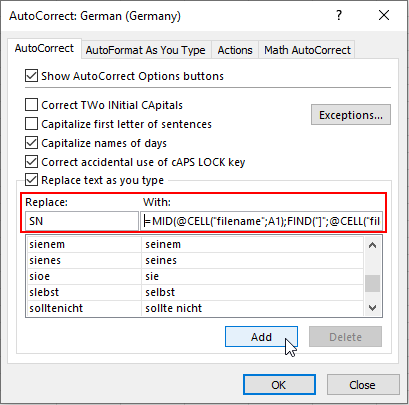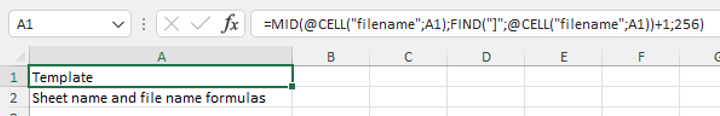# Easy-to-enter sheet and file name formulas

Here’s a great trick using autocorrect to easily enter a formula to show the name of the sheet or file. Set up once, use as often as you want with a simple two-letter entry.

I use these formulas a lot e.g., to create a flexible template sheet for my Excel models. When I copy the template and rename it, the sheet name (say in cell A1) automatically updates… fantastic!

How to set up
Here’s how to set it up for sheet name (a one-off exercise)...

1. Copy this formula if you have commas as separators
=MID(@CELL("filename",A1),FIND("]",@CELL("filename",A1))+1,256)
or this one if you have semi-colons as separators
=MID(@CELL("filename";A1);FIND("]";@CELL("filename";A1))+1;256)

2. Go to File, Options, Proofing, AutoCorrect Options…3. Under “Replace:” enter SN (for sheet name) and under “With:” paste the formula you copied in step 1, then “Add” and “OK” twice.To set up the filename formula follow the same steps but replace FN (for file name) with this formula if you have comma separators
=MID(@CELL("filename",A1),FIND("[",@CELL("filename",A1))+1,FIND(".xls",@CELL("filename",A1))-(FIND("[",@CELL("filename",A1))+1))

or this one if you have semi-colon separators
=MID(@CELL("filename";A1);FIND("[";@CELL("filename";A1))+1;FIND(".xls";@CELL("filename";A1))-(FIND("[";@CELL("filename";A1))+1))

How to useSimply go to a blank cell, type SN (space) and enter and you have your formula for sheet name!

Or type FN (space) and enter and you have your formula for file name!

(Technical tip: these formulas use the CELL function which only works if you have saved your file, because only then does it have a full path name).

Have fun with them!